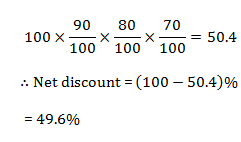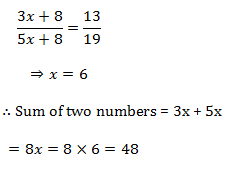# SSC Quantitative Aptitude Questions (Day-43)

Dear Aspirants, you can find the Quantitative Aptitude questions with detailed explanations for the SSC exams. Nowadays the competitive level of the exam has been increasing consistently. Due to the great demand for the government job, the level of the toughness reached greater. Candidates have to enhance the preparation process in order to drive in the right path. It doesn’t need to clear the prescribed cutoff. You must have to score good marks more than the cut off marks to get into the final provisional list. Here we have updating the Quantitative Aptitude questions with detailed explanations on a daily basis. You can practice with us and measure your level of preparation. According to that you can sculpt yourself in a proper way. SSC aspirants kindly make use of it and grab your success in your career.

Start Quiz

1) If 1/N = (√6 + √5)/(√6 – √5), then what is the value of N?

(a) 6 – √30

(b) 6 + √30

(c) 11 – 2√30

(d) 11 + 2√5

2) What is the value of positive square root of 69 + 28√5?

(a) 7 + 2√5

(b) 7 – 2√5

(c) 2 + 7√5

(d) 2 – 7√5

3) 311 + 312 + 313 + 314 is divisible by _____.

(a) 7

(b) 8

(c) 11

(d) 14

4) A boy added all natural numbers from 1 to 12, however he added one number twice due to which the sum becomes 80. What is the number which headded twice?

(a) 3

(b) 2

(c) 7

(d) 8

5) What smallest value must be added to 508, so that the resultant is a perfect square?

(a) 4

(b) 9

(c) 18

(d) 21

6) Raman is 25% more efficient than Aman. If Aman can complete a piece of work in 25 days, then Raman can complete the same work in how manydays?

(a) 12

(b) 15

(c) 16

(d) 20

7) A and B together can complete a work in 30 day. They started together but after 6 days A left the work and the work is completed by B after 36 moredays. A alone can complete the entire work in how many days?

(a) 45

(b) 90

(c) 60

(d) 120

8) The marked price of an article is 40% more than its cost price. If 10% discount is given, then what is the profit percentage?

(a) 10

(b) 20

(c) 26

(d) 32

9) What will be the net discount after giving three successive discounts of 10%, 20% and 30%?

(a) 50.4

(b) 49.6

(c) 45.3

(d) 48.4

10) The ratio of two numbers is 3:5. If both numbers are increased by 8, the ratio becomes 13:19. What is the sum of the two numbers?

(a) 32

(b) 48

(c) 40

(d) 72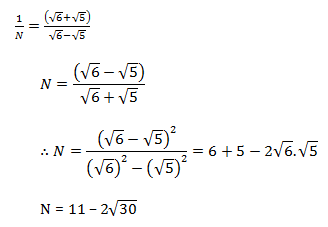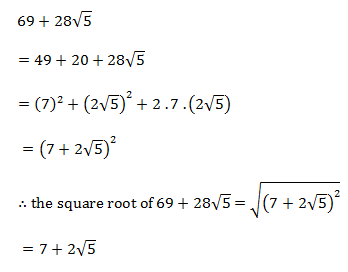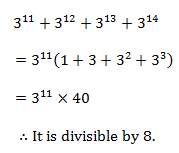Required Number = 80 – (1 + 2 + …+ 11 + 12)

= 80 – 78

= 2

508 + 21= 529

√529 = 23

Raman                       Aman

125                      100

Ratio of η⇒ 5     :           4

∴ Number of days taken by Raman = (4 × 25)/5 = 20 days

A + B ______ 30 days

1/5 work has been done by (A & B)

Now, 4/5 work is done by B in 36 days

∴ 1 work is done by B in (36 × 5)/4 = 45 days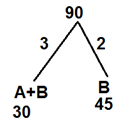So, efficiency of A = 1

So, A alone can do work in = 90/1 =90 days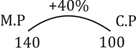After 10% discount on M.P

∴ S.P = 126

∴ Profit% = 26%